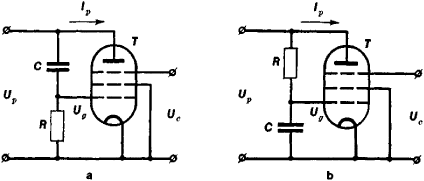Reactance Tube

reactance tube

[rē′ak·təns ‚tüb]
(electronics)
Vacuum tube operated in a way that it presents almost a pure reactance to the circuit.
McGraw-Hill Dictionary of Scientific & Technical Terms, 6E, Copyright © 2003 by The McGraw-Hill Companies, Inc.
The following article is from The Great Soviet Encyclopedia (1979). It might be outdated or ideologically biased.

Reactance Tube

a device consisting of an electron tube connected to a phase-shifting circuit; it has a controlled input reactance. The simplest phase-shifting circuit comprises a resistor R and a capacitor C connected in series (see Figure 1). If, as in Figure 1,a, one chooses 1/ωC ≫ R, then the phase of the voltage Ug on the control grid of the tube (usually a pentode) will lead the phase of the voltage Up on the plate by an angle of approximately 90°, and the phase of the current Ip in the plate circuit, which is practically the same as the phase of Ug, will lead Up by the same angle. If, as in Figure 1,b, 1/ ω CR, then there will be a phase lag instead of a lead. Such a phase shift of 90° between the voltage and the current is typical of the reactive elements in an electric circuit—a capacitor and an induction coil. Consequently, the impedance of the plate-cathode part of the tube (the input impedance of a reactance tube) is equivalent to a capacitive (Figure 1,a) or inductive (Figure 1,b) reactance. The value of the reactance can be varied within certain limits by controlling the tube’s plate current, for instance, by changing the bias voltage on the control or suppressor grid according to a given law.Figure 1. Diagrams of reactance tubes equivalent to (a) a capacitance and (b) an inductance: (Up) plate voltage, (Ug) grid voltage, (lp) plate current, (Uc) control voltage, (T) electron tube (pentode), (R) resistor and (C) capacitor in the phase-shifting circuit

Reactance tubes are used for automatic frequency control of electric oscillators, electronic tuning of the natural frequency in resonant circuits, and frequency modulation of oscillations. With the development of semiconductor electronics, reactance tubes in radio equipment have been almost universally replaced by devices with similar functions that use varicaps (varactors) and, more rarely, transistors.

REFERENCES

Artym, A. D. Teoriia i metody chastotnoi moduliatsii. Moscow-Leningrad, 1961.
Gonorovskii, I. S. Radiotekhnicheskie tsepi i signaly, 2nd ed. Moscow, 1971.
M. V. KAPRANOV